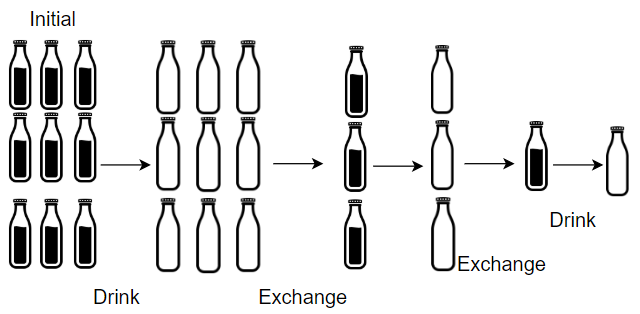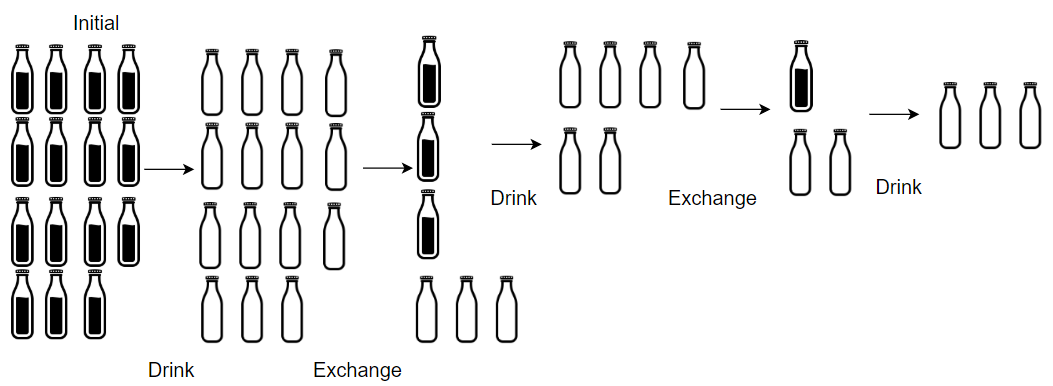GeetCode Hub

Given numBottles full water bottles, you can exchange numExchange empty water bottles for one full water bottle.

The operation of drinking a full water bottle turns it into an empty bottle.

Return the maximum number of water bottles you can drink.

Example 1:Input: numBottles = 9, numExchange = 3
Output: 13
Explanation: You can exchange 3 empty bottles to get 1 full water bottle.
Number of water bottles you can drink: 9 + 3 + 1 = 13.

Example 2:Input: numBottles = 15, numExchange = 4
Output: 19
Explanation: You can exchange 4 empty bottles to get 1 full water bottle.
Number of water bottles you can drink: 15 + 3 + 1 = 19.

Example 3:

Input: numBottles = 5, numExchange = 5
Output: 6

Example 4:

Input: numBottles = 2, numExchange = 3
Output: 2

Constraints:

• 1 <= numBottles <= 100
• 2 <= numExchange <= 100

class Solution { public int numWaterBottles(int numBottles, int numExchange) { } }